## Tuesday, June 23, 2015

### Update to rerootingMethod for ancestral state reconstruction to permit polytomies

A phytools user recently reported that the ancestral character estimation method for discrete traits in phytools, `rerootingMethod`, does not work for trees with polytomies. This is in fact correct - although I had long forgotten that this was the case.

The function `rerootingMethod` uses the approach of Yang et al. (1995) to compute the marginal ancestral states by re-rooting the tree at all internal nodes. For instances in which some states at the tips are uncertain, it can also re-root at the tip to compute empirical Bayesian posterior probabilities for the leaves as well. The function is less relevant now that `ace` in the ape package also can compute the marginal ancestral states. (Although, as far as I know, `ace` can also not handle polytomies.) `rerootingMethod` stays somewhat relevant only by virtue of being able to compute posterior probabilities at tips, and for handling uncertainty at tip states (two sides of the same coin, I guess).

It was fairly straightforward to update `rerootingMethod` to handle polytomies, and the code is posted here. The way that this current version works is pretty simple. It just first takes the input tree, checks for polytomies using `is.binary.tree` in the ape package, resolves polytomies randomly with branches of zero length using `multi2di`, estimates ancestral states for all internal nodes (& tips, if `tips=TRUE`), and the uses the phytools function `matchNodes` to back-translate the reconstructed nodes in the resolved tree to the original, input tree. Note that although it is doing something here that `ace` does not do - the code internally uses (modified code from) `ace`.

Here - we can check it out by simulating a tree with some polytomies, generating trait data for tips & nodes, and then reconstructing ancestral states on the tree:

``````library(phytools)
dt<-rtree(n=26)
dt\$tip.label<-LETTERS
## set some of the internal branches
ii<-which(dt\$edge[,2]>Ntip(dt))
dt\$edge.length[sample(ii,4)]<-0
mt<-di2multi(dt)
plotTree(mt)
``````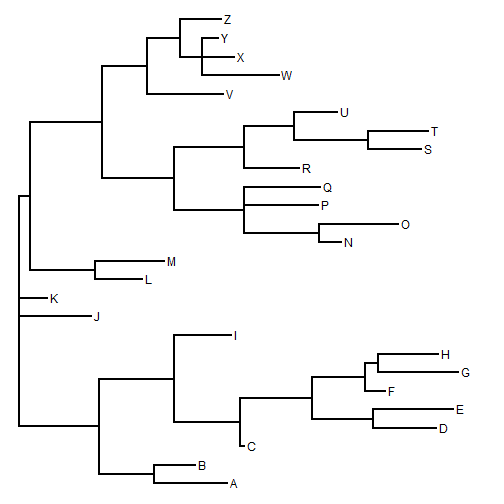Next let's simulate a character up the tree:

``````Q<-matrix(c(-1,0.5,0.5,
0.5,-1,0.5,0.5,0.5,-1),3,3,
dimnames=list(letters[1:3],letters[1:3]))
st<-sim.history(mt,Q)
``````
``````## Done simulation(s).
``````
``````x<-st\$states
y<-getStates(st,"nodes")
``````

Now, we can reconstruct & plot ancestral states using `ace` on the bifurcating tree; and `rerootingMethod` on the bi- and on the multifurcating trees. If the methods are working properly then the reconstructions will be the same - although in the bifurcating node there will be additional nodes with states identical to other nodes from which they are separated by branches of zero length.

``````## first ace
fit1<-ace(x,mt,type="discrete",model="ER") ## doesn't work
``````
``````## Error in ace(x, mt, type = "discrete", model = "ER"): "phy" is not rooted AND fully dichotomous.
``````
``````fit2<-ace(x,dt,type="discrete",model="ER") ## doesn't work
``````
``````## Error in ace(x, dt, type = "discrete", model = "ER"): some branches have length zero or negative
``````
``````## set zero-length branches to be 1/1000000 total tree length
dst<-dt
dst\$edge.length[dst\$edge.length==0]<-max(nodeHeights(dt))*1e-6
fit3<-ace(x,dst,type="discrete",model="ER")
fit3
``````
``````##
##     Ancestral Character Estimation
##
## Call: ace(x = x, phy = dst, type = "discrete", model = "ER")
##
##     Log-likelihood: -25.3342
##
## Rate index matrix:
##   a b c
## a . 1 1
## b 1 . 1
## c 1 1 .
##
## Parameter estimates:
##  rate index estimate std-err
##           1   0.3278  0.1042
##
## Scaled likelihoods at the root (type '...\$lik.anc' to get them for all nodes):
##          a          b          c
## 0.82837257 0.09781827 0.07380916
``````

Now with the new version of `rerootingMethod`:

``````packageVersion("phytools")
``````
``````##  '0.4.57'
``````
``````fit4<-rerootingMethod(mt,x,model="ER")
fit4
``````
``````## \$loglik
##  -25.33421
##
## \$Q
##            a          b          c
## a -0.6556595  0.3278298  0.3278298
## b  0.3278298 -0.6556595  0.3278298
## c  0.3278298  0.3278298 -0.6556595
##
## \$marginal.anc
##               a          b            c
## 27 0.8283833959 0.09781145 0.0738051507
## 28 0.2121485528 0.69489845 0.0929529991
## 29 0.0532939602 0.91028552 0.0364205221
## 30 0.0627113681 0.88952034 0.0477682947
## 31 0.0132238730 0.97569370 0.0110824277
## 32 0.3870898035 0.30541903 0.3074911711
## 33 0.4035347684 0.15548939 0.4409758369
## 34 0.5865138808 0.07266794 0.3408181834
## 35 0.3530969369 0.06523786 0.5816652004
## 36 0.7045263050 0.13922658 0.1562471186
## 37 0.1288634178 0.06087131 0.8102652730
## 38 0.3401006710 0.49952777 0.1603715570
## 39 0.4129576403 0.32707964 0.2599627226
## 40 0.1266883464 0.35550347 0.5178081798
## 41 0.0553700550 0.21371716 0.7309127832
## 42 0.8088376946 0.10680265 0.0843596595
## 43 0.8570510600 0.08945954 0.0534894017
## 44 0.6274100032 0.30199393 0.0705960682
## 45 0.0836944308 0.86315981 0.0531457619
## 46 0.0119373448 0.97893201 0.0091306462
## 47 0.0006664523 0.99874487 0.0005886784
``````

If we plot both of these on our trees in turn, we should see that the marginal reconstructions (excepting the small deviation that was required to give our tree non-zero branch lengths throughout) should be equal:

``````## first ace
plotTree(dt,offset=0.5)
nodelabels(pie=fit3\$lik.anc)
tiplabels(pie=to.matrix(x,seq=letters[1:3]),cex=0.5)
``````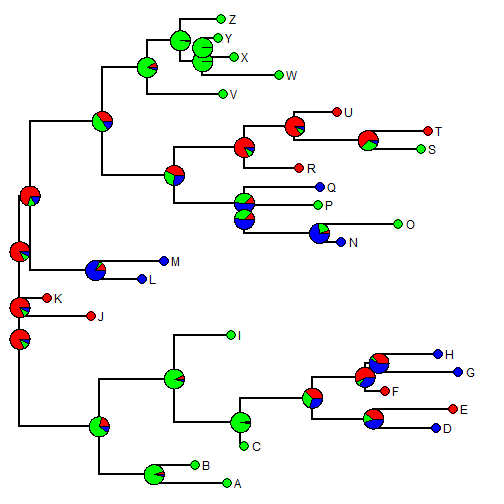``````## now phytools
plotTree(mt,offset=0.5)
nodelabels(pie=fit4\$marginal.anc)
tiplabels(pie=to.matrix(x,seq=letters[1:3]),cex=0.5)
``````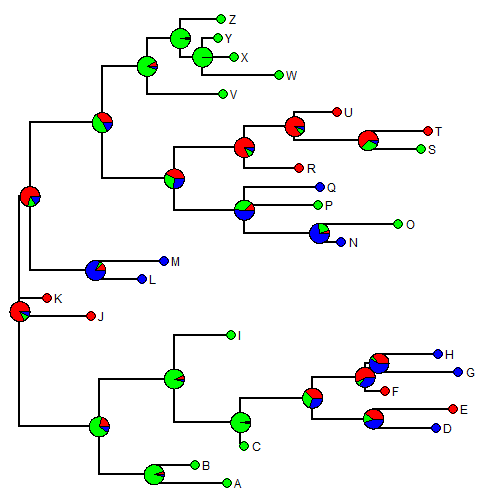Finally, for fun let's overlay the true, known states to see how close (or off) we were:

``````plotSimmap(st,colors=setNames(c("red","green","blue"),letters[1:3]),
offset=0.5)
nodelabels(pie=fit4\$marginal.anc)
tiplabels(pie=to.matrix(x,seq=letters[1:3]),cex=0.5)
``````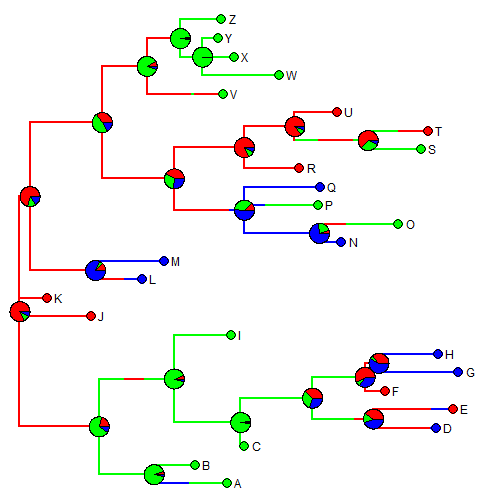That's it.

1.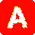I am unable to run the rerootingMethod, despite it having the same format input of 'x':

Error in `colnames<-`(`*tmp*`, value = c("a", "c")) :
attempt to set 'colnames' on an object with less than two dimensions

Any suggestions? Thanks

1.Hi Alex. What is your object x? It should be a vector of type character (or a factor) with names corresponding to the species names in the tree.

2.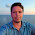Hi Liam,
I’m working on adding evolution of a discrete character to a phylogeny. First I am attempting to model ER, SYM, and ARD using rerootingMethod. ER and ARD seem to work properly, with ARD being a better fit. SYM, however, produces the error “In log(comp[1:M + N]) : NaNs produced”. I suspect from your post here (http://blog.phytools.org/2016/05/more-on-plot-method-for-object-class.html) it has to do with parameters versus tips, but in that case, why does ARD seem to work? Furthermore, when I move onto using make.simmap to see the (code: SYMtrees <- make.simmap(tree,character,model="SYM",nsim=1000,q="mcmc"), the resulting sectors in the node piecharts are all equal, and the same process for ARD produces NaNs but with a sensible output. Does this suggest that the character and phylogeny will only support the simpler ER model? Is there something else I am missing? Thank you!
-Bob

3.dst<-tree
> dst\$edge.length[dst\$edge.length==0]<-max(nodeHeights(tree))

How can this be used on a class of multi.phylo (I have over 3000 posterior trees from a Bayes anaylsis).

I am trying to run SIMMAP but it keeps giving me this error " Error in xx[tree\$tip.label, ] : subscript out of bounds"

I assume that means I need to use the code you used above to set non zero lengths to 1/1000 however when I try to use the code above I get this error " Error in nodeHeights(ntrees) : tree should be an object of class "phylo"."

However, When I use 'is.binary.multiphylo' I get "true" for all 3000 trees. Any help with this would be greatly appreciated.

4.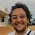This comment has been removed by the author.

Note: due to the very large amount of spam, all comments are now automatically submitted for moderation.